# Difference between revisions of "Wreath product"

The wreath product of two groupsandis constructed in the following way. Let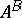be the set of all functions defined onwith values in. With respect to componentwise multiplication, this set is a group which is the complete direct product of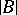copies of(denotes the number of elements in);acts onas a group of automorphisms in the following way: if,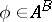, thenfor. With respect to this operation, one can form the semi-direct productofand, that is, the set of all pairs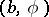, where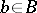,, with multiplication operation given by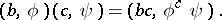The resulting groupis called the Cartesian (or complete) wreath product ofand, and is denoted by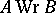(or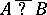, a denotation of Ph. Hall). If instead ofone takes the smaller group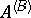consisting of all functions with finite support, that is, functions taking only non-identity values on a finite set of points, then one obtains a subgroup ofcalled the wreath product (direct wreath product, discrete wreath product) ofand; it is denoted by(or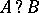). Both wreath products are widely used for constructing various examples of groups.